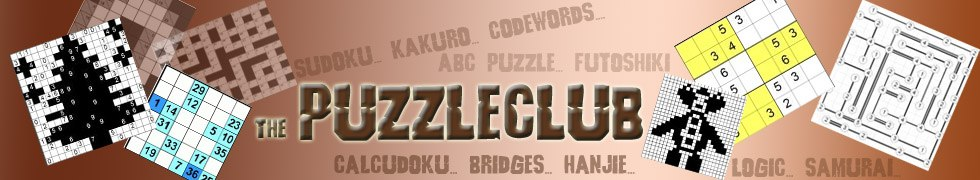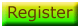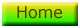# Killer Sudoku Strategy

Killer Sudoku is a fun variant of the standard sudoku puzzle. All the rules remain in place with regard the rows, columns and 3 x 2 or 3 x 3 squares containing the numbers 1 - 6 or 1 - 9 once and once only. However the twist is the grid is split into various shapes, and for each shape a number is given which corresponds to the sum of the cells that compose that shape. And no starting numbers are given to help you out! Here are some strategies to use to solve the puzzle:

## 1 - Look for cells with the least combinations

The best way to get started is to look for the cells that can have the least possible values from the start. In this example we use parts of a 3 x 2 puzzle, therefore the numbers used are from 1 to 6. Look at this portion of a grid below: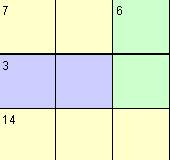Here we can see there is a '3' composed of two cells. We know this must be '2' and '1' or '1' and '2', so mark it accordingly using pencil marks. This gives us: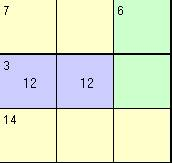## 2 - Use your pencilmarks to deduce values elsewhere

Often you don't need to know where a number goes for sure to work out what another number must be. Let's see this here by applying the first rule to the bottom left of the example. We see a run of '14' composed of three cells. We know that this can only be composed of 6, 5, 3, so let's mark that accordingly on the grid: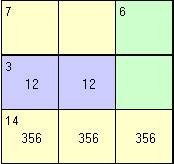Now despite not knowing exactly where the 1,2,3,5,6 go in this 3 x 2 square, we know that those five cells must contain those numbers in some order. We can now apply the rule that each 3 x 2 must contain 1 - 6 once exactly to place the top right value with certainty - it must be a '4'. Thus we place it in the grid: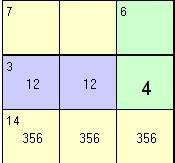This is probably the logical technique combined with rule application you will use most often in killer sudoku - for instance once you know the cells where a number must go in a row or column even if you cannot isolate exactly which, you then know it cannot go anywhere else in that row or column.

## 3 - Apply rule 2 again!

Once you have used the second rule to place a number, apply it again! Here's an example of how powerful this technique is. Let's carry on from where we were, and show the rest of the row that contains the '4' we have now placed: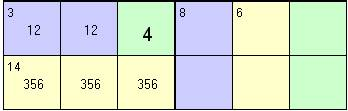Now, we know the 1,2,4 are placed in the first three cells of the row. Therefore we know that the three empty cells at the right of the row must contain one of 3,5,6. But we can go further! The cell containing the '6' cannot contain a '6', meaning it must contain a '3' or '5'. But it cannot contain a '3' because then the sum for '6' would be 3, 3 which is against the rules. Therefore this square must contain a '5'! Thus we place it, and move the cursor to the '8', as we are about to place that next!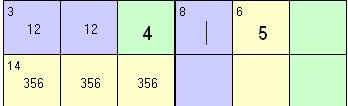We now know the '8' cannot contain a '5' as the '5' is already placed in the 3 x 2 now. It can't be '4', '4' (repetition) therefore it must be '6' and '2'. But since we know the '2' for the row must be in cell one or two, we know that it must be '6', and thus we mark it accordingly, and also write in the '3' at the right edge as that's the only possible value left for that cell. Similarly we can place a '4' in the bottom right for the same reason.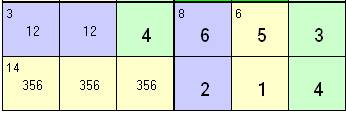Good luck! By looking for cells with few combinations throughout a puzzle and working out what that must mean for the rest of the puzzle, you will have taken an important step towards successfully solving a killer sudoku puzzle.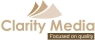©   |     |